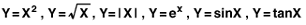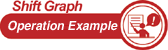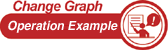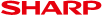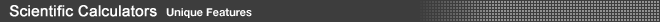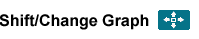These functions help students understand the relationship between an equation and its graph.• Shift Graph Shift the location of the graph. Notice how the graph changes and how it is reflected in the equation.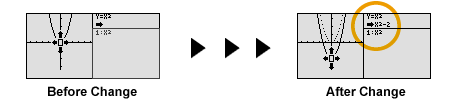Built-in functions: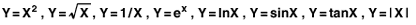• Change Graph Change the scope of the graph. Notice how the graph changes and how it is reflected in the equation.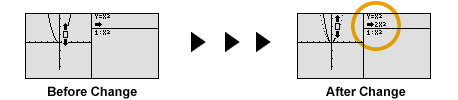Built-in functions: